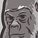Announcements
Applications are open for the 2024 Qlik Luminary Program. Apply by December 15 here.
cancel
Showing results for
Did you mean:Contributor III

Hello,

I am pretty new at Qlik Sense and have a question where you might be able to support.

I loaded an excel file in Qlik Sense with several columns: ColumnA/ColumnB/ColumnC/ColumnD/ColumnE/ColumnF/ColumnG

I want to add three columns to the table above:

ColumnH:

if ColumnA IS empty, ColumnH is "Value1"

If ColumnA IS NOT empty AND ColumnB = "N", ColumnH is "Value2"

If ColumnA IS NOT empty AND ColumnB = "Y" AND ColumnC IS empty, ColumnH is "Value3"

If ColumnA IS NOT empty AND ColumnB = "Y" AND ColumnC ="2", ColumnH is "Value4"

If ColumnA IS NOT empty AND ColumnB = "Y" AND ColumnC ="3", ColumnH is "Value5"

If ColumnA IS NOT empty AND ColumnB = "Y" AND ColumnC ="4", ColumnH is "Value6"

ColumnI:

If ColumnB = "Y" AND ColumnD IS empty, ColumnI is "Value7"

If ColumnB = "Y" AND ColumnD contains "Root", ColumnI is "Value8"

If ColumnB = "Y" AND ColumnD NOT contains "Root", ColumnI is "Value9"

ColumnJ:

ColumnJ = ColumnE + "_" + ColumnF + "_" + ColumnG

May I ask how would it work in the Data load editor?

Best regards,
Rubén

Labels (3)

• ### Qlik Sense

2 Solutions

Accepted SolutionsPartner - Specialist

ColumnA,
ColumnB,
ColumnC,
ColumnD,
ColumnE,
ColumnF,
ColumnG,
If(Len(Trim(ColumnA))=0,'Value1',If(ColumnB='N','Value2',If(Len(Trim(ColumnC))=0,'Value3',Pick(ColumnC-1,'Value4','Value5','Value6')))) AS ColumnH,
If(ColumnB = 'Y',If(Len(Trim(ColumnD))=0,'Value7',If(ColumnD='Root','Value8','Value9'))) AS ColumnI,
ColumnE&'_'&ColumnF&'_'&ColumnG AS ColumnJ
From
...

Hope this helps.

MauritzPartner - Specialist

Sorry, I misunderstood your requirement. You can use SubStringCount.

If(ColumnB = 'Y',If(Len(Trim(ColumnD))=0,'Value7',If(SubStringCount(ColumnD,'Root')>0,'Value8','Value9'))) AS ColumnI

Let me know if you still have problems.

Regards,

Mauritz

3 RepliesPartner - Specialist

ColumnA,
ColumnB,
ColumnC,
ColumnD,
ColumnE,
ColumnF,
ColumnG,
If(Len(Trim(ColumnA))=0,'Value1',If(ColumnB='N','Value2',If(Len(Trim(ColumnC))=0,'Value3',Pick(ColumnC-1,'Value4','Value5','Value6')))) AS ColumnH,
If(ColumnB = 'Y',If(Len(Trim(ColumnD))=0,'Value7',If(ColumnD='Root','Value8','Value9'))) AS ColumnI,
ColumnE&'_'&ColumnF&'_'&ColumnG AS ColumnJ
From
...

Hope this helps.

MauritzContributor III
Author

Hello @Mauritz_SA ,

It works almost perfect but I still have one mistake.

In the following formula

If(ColumnB = 'Y',If(Len(Trim(ColumnD))=0,'Value7',If(ColumnD='Root','Value8','Value9'))) AS ColumnI,

Column D contains (or not) the word "Root" but it not alone. It means, the content of the cell could be "Root cause: Supplier" or "Root cause: Manufacturing".  At the moment, with the formula above, it displays in ColumnI either Value7 or Value9 but never Value8. Can you maybe support here? I tried putting *Root* and it is unfortunately not working.

Thank you.

Best regards,
RubénPartner - Specialist

Sorry, I misunderstood your requirement. You can use SubStringCount.

If(ColumnB = 'Y',If(Len(Trim(ColumnD))=0,'Value7',If(SubStringCount(ColumnD,'Root')>0,'Value8','Value9'))) AS ColumnI

Let me know if you still have problems.

Regards,

MauritzTags
Community Browser